/10

test exam

test exam

1 / 10

1) Take a look at the shapes below which are similar in some way: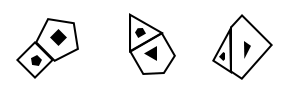Select the image which is related in a similar way to the shapes above.

2 / 10

2) Take a look at the shapes below which are similar in some way:Select the image which is related in a similar way to the shapes above.

3 / 10

3) Take a look at the shapes below which are similar in some way:Select the image which is related in a similar way to the shapes above.

4 / 10

4) Take a look at the shapes below which are similar in some way:Select the image which is related in a similar way to the shapes above.

5 / 10

5) Take a look at the shapes below which are similar in some way: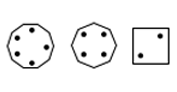Select the image which is related in a similar way to the shapes above.

6 / 10

6) Take a look at the shapes below which are similar in some way: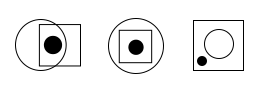Select the image which is related in a similar way to the shapes above.

7 / 10

7) Take a look at the shapes below which are similar in some way: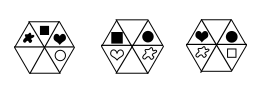Select the image which is related in a similar way to the shapes above.

8 / 10

8) Take a look at the shapes below which are similar in some way: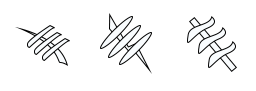Select the image which is related in a similar way to the shapes above.

9 / 10

9) Take a look at the shapes below which are similar in some way: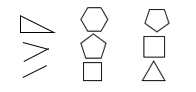Select the image which is related in a similar way to the shapes above.

10 / 10

10) Take a look at the shapes below which are similar in some way:Select the image which is related in a similar way to the shapes above.